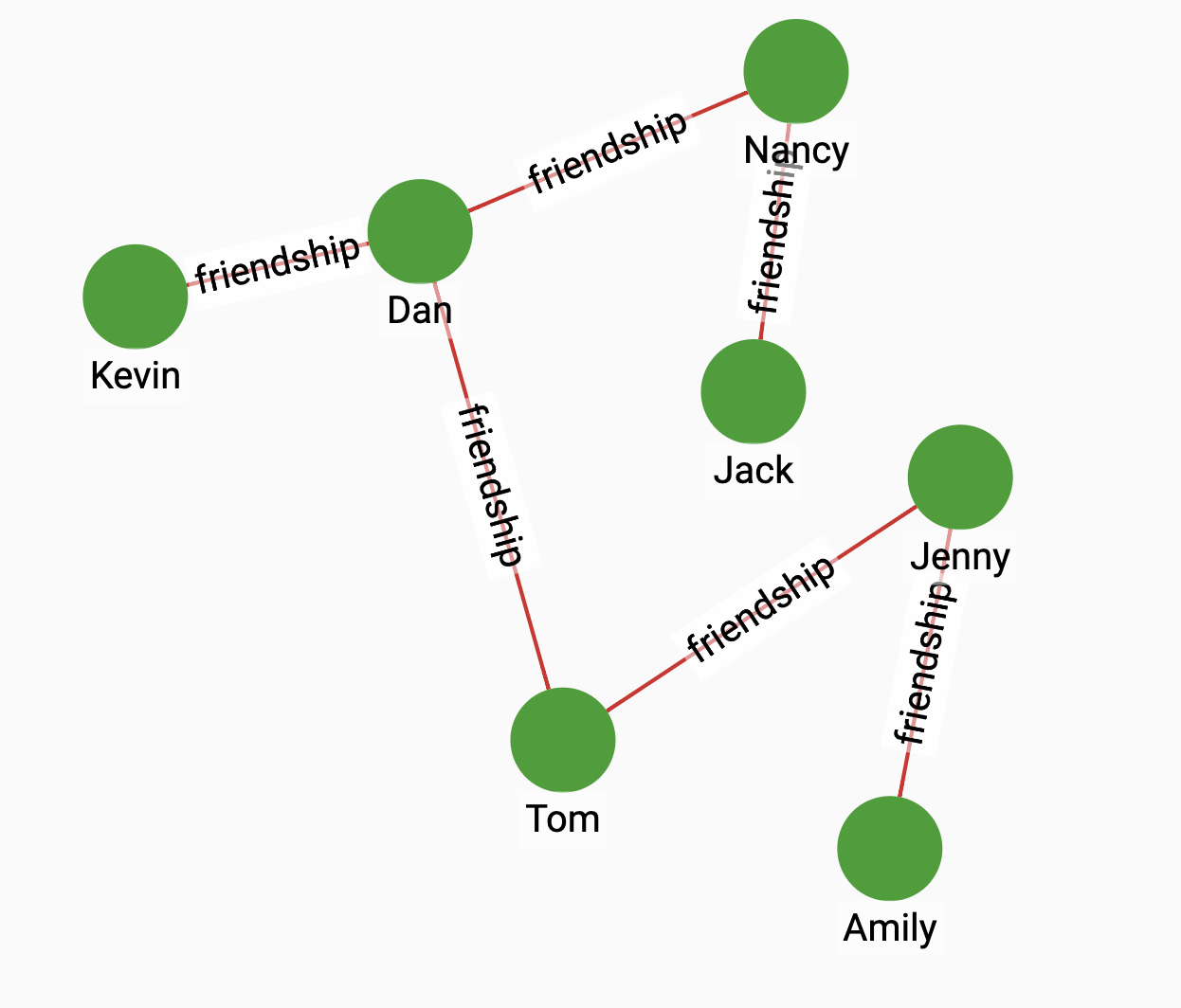# Eigenvector Centrality

Eigenvector centrality (also called eigencentrality or prestige score) is a measure of the influence of a vertex in a network. Relative scores are assigned to all vertices in the network based on the concept that connections to high-scoring vertices contribute more to the score of the vertex in question than equal connections to low-scoring vertices. A high eigenvector score means that a vertex is connected to many vertices who themselves have high scores.

## Specification

```CREATE QUERY tg_eigenvector_cent(SET<STRING> v_type, SET<STRING> e_type,
INT maxIter = 100, FLOAT convLimit = 0.000001, INT top_k = 100,
BOOL print_accum = True, STRING result_attr = "", STRING file_path = ""
)```

### Time complexity

The algorithm has a time complexity of O(E*k), where E = number of edges, k = number of iterations. The number of iterations is data-dependent, but the user can set a maximum. Parallel processing reduces the time needed for computation.

## Parameters

Name Description Data type

`v_type`

Vertex types to assign scores to.

`SET<STRING>`

`e_type`

Edge types to traverse.

`SET<STRING>`

`maxIter`

Maximum number of iteration.

`INT`

`convLimit`

The convergence limit.

`FLOAT`

`top_k`

The number of vertices with the highest scores to return.

`INT`

`print_accum`

If true, print results to JSON output.

`BOOL`

`result_attr`

If not empty, save the score of each vertex to this attribute.

`STRING`

`file_path`

If not empty, print results in CSV to this file.

`STRING`

The I

## Example

Suppose we have the following graph:Running the algorithm on the graph will show that Dan has the highest centrality score.

• Query

• Result

``RUN QUERY tg_eigenvector_cent(["person"], ["friendship"])``
``````{
"error": false,
"message": "",
"version": {
"schema": 2,
"edition": "enterprise",
"api": "v2"
},
"results": [{"top_scores": [
{
"score": 0.59598,
"Vertex_ID": "Dan"
},
{
"score": 0.50223,
"Vertex_ID": "Jenny"
},
{
"score": 0.44381,
"Vertex_ID": "Tom"
},
{
"score": 0.28786,
"Vertex_ID": "Nancy"
},
{
"score": 0.24085,
"Vertex_ID": "Kevin"
},
{
"score": 0.20296,
"Vertex_ID": "Amily"
},
{
"score": 0.11633,
"Vertex_ID": "Jack"
}
]}]
}``````